## Ramanujan Type Series

Ramanujan's remarkable series for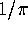include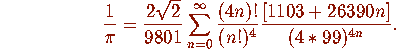This series adds roughly eight digits per term. Gosper in 1985 computed 17 million terms of the continued fraction for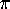using this.

Such series exist because various modular invariants are rational (which is more-or-less equivalent to identifying those imaginary quadratic fields of class number 1).

The Chudnovskys' series with d = -163 is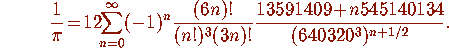Quadratic versions correspond to class number two imaginary quadratic fields. The largest example has d = -427 and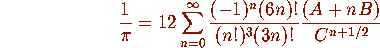whereThis series adds roughly 25 digits per term. These series are of the form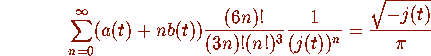where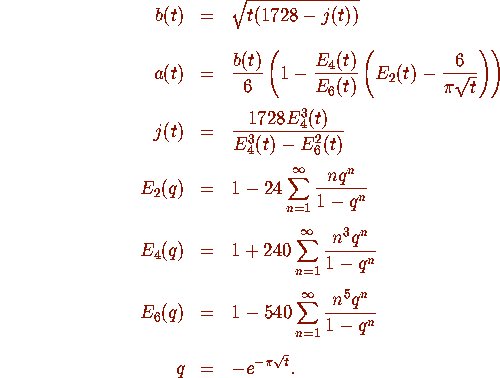Here t is the appropriate discriminant, j is the ``absolute invariant'', and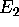,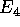and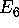are Eisenstein series. There is an unlimited number of such series with increasingly more rapid convergence. The price is that one must deal with more complicated algebraic irrationalities.

A class number p field will involve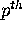degree algebraic integers as the constants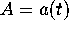,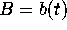and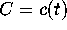in the series.

The largest class number three example with d = -907 gives 37 or 38 digits per term.

The largest class number four example with d = -1555 is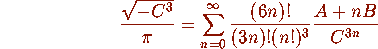where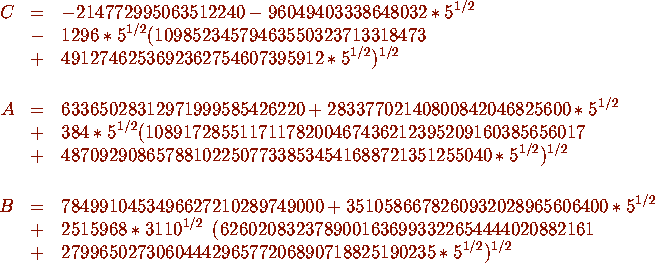This gives 50 additional digits per term.

this is discussed in Class number three Ramanujan type series for 1/Pi in J. Comp. and Applied Math. 46 (1993) 281-290.

Deriving the SeriesThe absolute invariant, and so the coefficients A, B, and C satisfy polynomial equations of known degree and height.Thus the problem of determining the coefficients of each series reduces to algebra and can be entirely automated.From the expressions for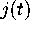,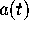,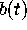it is easy to compute their values to several hundred digits.The lattice basis reduction algorithm now provides the minimal polynomials for each quantity.In addition, a higher precision calculation actually provides a proof of the claimed identity.This last step requires knowing a priori bounds on the degrees and heights.